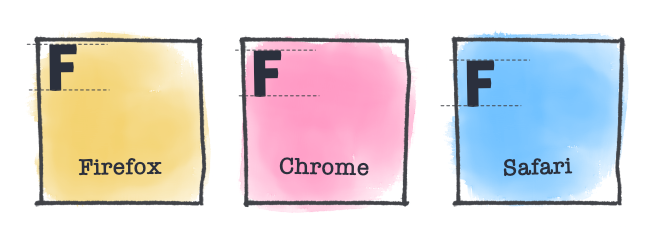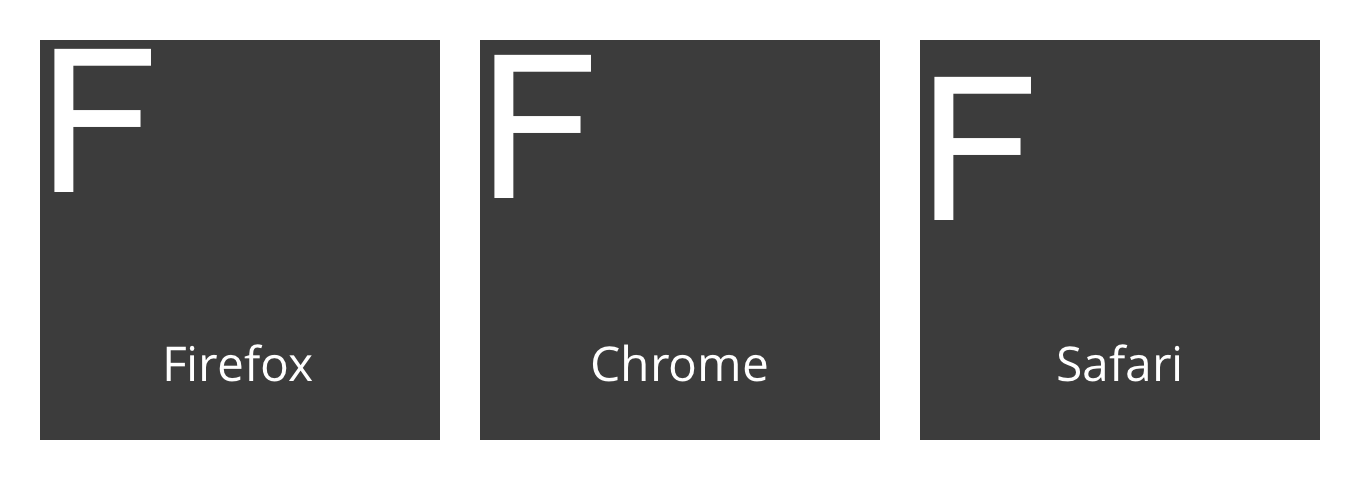# Cross-Browser Alignment of the Canvas fillText Draw Call

If we use the HTML5 canvas `fillText` API and compare output from various browsers we see that the draw offset differs slightly. In this quick tutorial we’ll use a snippet to determine this offset and then correct the draw position.## Differences

The article banner is just an impression, but reality is not far off. We can see the actual difference in draw offset below, it’s just a few pixels, but it’s there.When drawing image data on the client, like for example with Doka Image Editor, we want every browser to deliver the same output.

## Solution

We can solve this issue by drawing an “F” character to a canvas element and then looking for the first horizontal pixel and the first vertical pixel where it was drawn.

Let’s draw a big “F”. A size of `100px` should suffice. We want to align our text from the top, so we set `textBaseline` top `"top"`.

``````// create the canvas
const canvas = document.createElement('canvas');
canvas.width = 32;
canvas.height = 32;

// do the drawing
const ctx = canvas.getContext('2d');
ctx.font = `100px sans-serif`;
ctx.fillStyle = '#fff'; // color of our F
ctx.textBaseline = 'top'; // alignment
ctx.fillText('F', 0, 0); // finally draw the F
``````

This will draw the “F” in the top left corner. We don’t need to know everything about this “F”. We’re only interested in the top and left pixels, that’s why our canvas is so small. See below for the result.

Now we need to find out where it was drawn. We’ll use the canvas `getImageData` method to get an array of pixel values to work with. Each index in the array represents a color value. A pixel consists of 4 colors, as our canvas is 32 pixels width, each “line” of the image consist of 4 * 32 indexes.

We’ll traverse the image vertically along the right most edge, and horizontally along the bottom. That should result in us bumping into our “F”.

``````
// get pixel data so we can find the white pixels
const data = ctx.getImageData(0, 0, 32, 32).data;

// "pixel" position p in data
let p;

// find x offset (bottom row of pixels)
let from = data.length - (32 * 4);

// increase by 4 to step through the data per pixel instead of per color value
for (p = from; p < data.length; p += 4) {
// stop when we find a value other than 0
if (data[p]) break;
}

// calculate the x offset based on the current pixel
const x = (p - from) / 4;

// find y offset (right column of pixels)
from = 31 * 4;
for (p = from; p < data.length; p += 32 * 4) {
if (data[p]) break;
}

// calculate the y offset based on the current pixel
const y = (p - from) / (32 * 4);

// log the position to the console (it's there, go take a look)
console.log(x, y);

``````

Now we have the browser offset based on a `100px` font size. We can convert this to another font size by multiplying our font size by `0.01` and then multiplying by our browser offset.

We’ll further invert the browser offset to compensate for the discrepancy among browsers.

Our compensated font offset for a `16px` font:

``````const xOffset = -x * (16 * .01);
const yOffset = -y * (16 * .01);
``````

If we use these offsets in our `fillText` function it’ll render the text at the correct position on each browser.

To prevent text from being drawn too far to the left we can set a default offset. A value like `{ x: 8, y: 4 }` should be fine.

``````const xOffset = -x * (16 * .01) + 8;
const yOffset = -y * (16 * .01) + 4;
``````

The uncorrected output, this should (shouldn’t but that’s reality) be different for each browser:

With correction applied, the image below should the same on every browser:

## Conclusion

By measuring the actual draw position of each browser we can determine a new position to draw to and align text positions accross multiple browsers. Note that so far this has been tested on Safari, Chrome, and Firefox, there are other browsers out there that might yield different results.

If you have any questions, or find a bug, let me know on Twitter.Rik Schennink

Web enthusiast

to pqina.nl